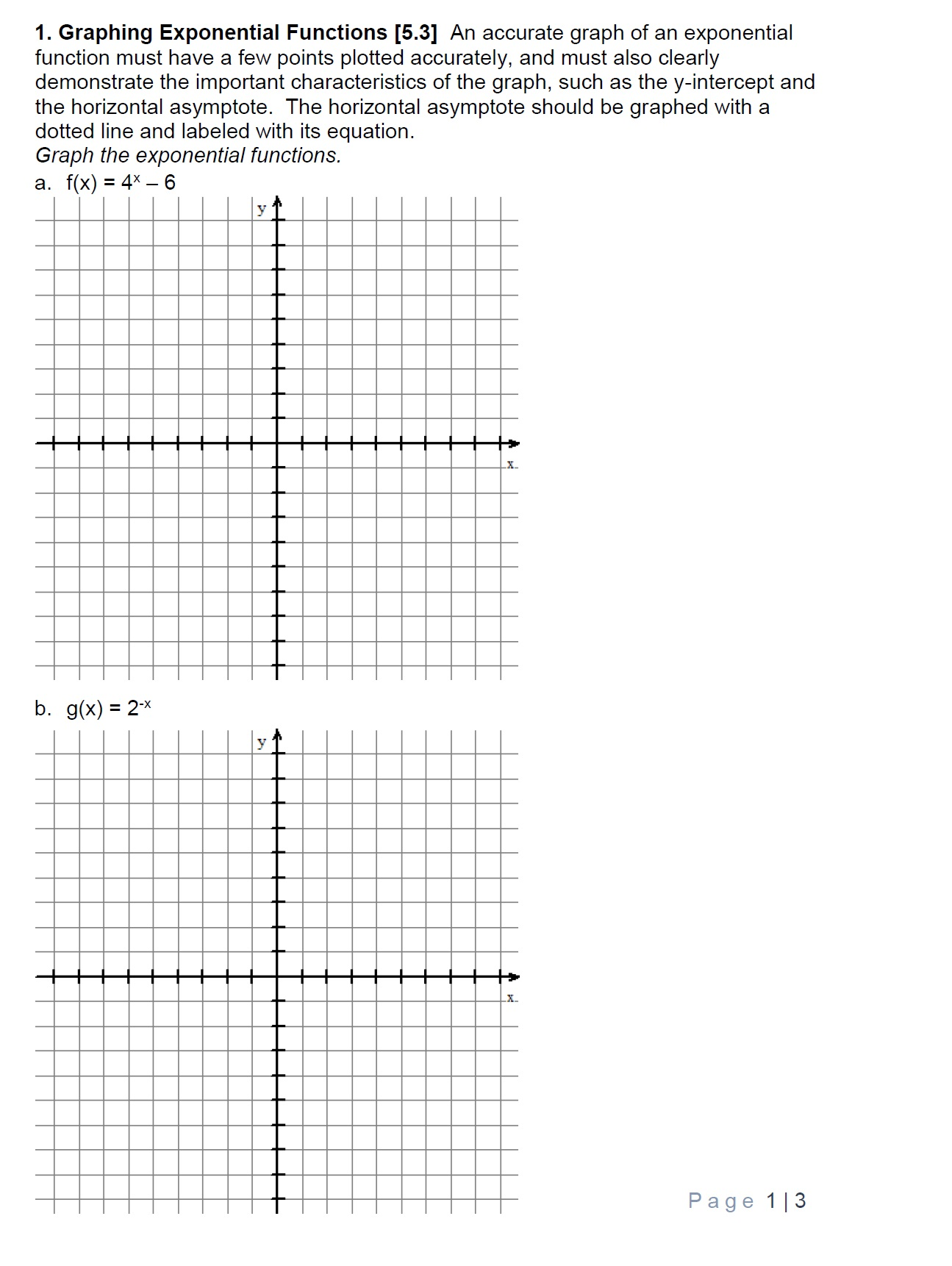# 1. Graphing Exponential Functions [5.3] An accurate graph of an exponentialfunction must have a few points plotted accurately, and must also clearlydemonstrate the important characteristics of the graph, such as the y-intercept andthe horizontal asymptote. The horizontal asymptote should be graphed with adotted line and labeled with its equation.Graph the exponential functions.a. f(x)=4"-6b. g(x)-2xage 1 13

Question
17 viewshelp_outlineImage Transcriptionclose1. Graphing Exponential Functions [5.3] An accurate graph of an exponential function must have a few points plotted accurately, and must also clearly demonstrate the important characteristics of the graph, such as the y-intercept and the horizontal asymptote. The horizontal asymptote should be graphed with a dotted line and labeled with its equation. Graph the exponential functions. a. f(x) =4"-6 b. g(x)-2x age 1 13 fullscreen
check_circle

Step 1

Given information :

a) The given function is f(x)=4^x-6.

b) The given function is g(x)=2^(-x).

Concept Used:

For x-intercept, substitute y=0 and solve for x.

For y-intercept, substitute x=0 and solve for y.

If the function is exponential function is of the form

Step 2

then the horizontal asymptotes is y=k.

Calculate:

First, we have to find the few points to draw the graph.

Step 3

For x-intercept, substitute f(x)=0 ...

### Want to see the full answer?

See Solution

#### Want to see this answer and more?

Solutions are written by subject experts who are available 24/7. Questions are typically answered within 1 hour.*

See Solution
*Response times may vary by subject and question.
Tagged in

### Other